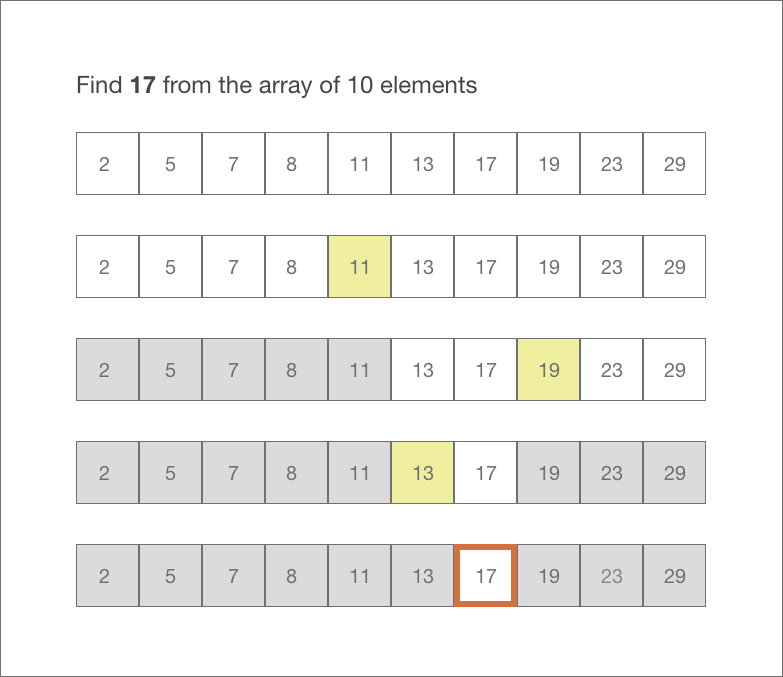# Binary Search Algorithm in JavaScript## Binary Search Algorithm

Binary search is an algorithm to find an element in a sorted array. Binary search works by repeatedly dividing the array into half until you’ve narrowed down the possible locations to just one.

In Binary search, we start with the array of sorted elements. We check if the value of the middle element in the array is equal to, greater to, or less than the value of the element to be searched. If the value of the mid-point is equal to the element to be searched, we have found the element.

If the value of the element to be searched is less than the mid-point, we narrow the interval of the array to the lower half in the next iteration. Else we consider the upper half. We repeat the process until the element is found. Thus, we ignore half of the elements in each iteration.In the example above, we want to find the element 17 from the array of 10 elements.

1. We start by finding the middle element which is 11. Now since 17 is greater than 11, we narrow the interval of the array and consider only the elements greater than 11 `[13, 17, 19, 23, 29]` in the next iteration.
2. 19 is the middle element in the second iteration and since 17 is smaller than 19, we narrow the interval to the lower half `[13, 17]` in the next iteration.
3. We continue iterating until the mid-point equals 17.

The time complexity of the Binary Search Algorithm is O(log N).

## Implementation of the Binary Search Algorithm in JavaScript.

The Iterative approach for Binary Search Algorithm:

```function binarySearchIterative(arr, value ){
var mid, left, right;
left = 0;
right = arr.length-1;

while(left<=right){
mid = parseInt((left+right)/2);
if(value === arr[mid]){
return "Found";
}
else if(value < arr[mid]){
right = mid-1;
}
else {
left = mid+1;
}
}
}

var arr = [2,3,5,7,9,12,17,20];
binarySearchIterative(arr, 17);```

The Recursive approach for Binary Search Algorithm:

```function binarySearch(arr, value ){
var mid, left, right;

if(arr.length === 1 && arr!==value){
}

mid = parseInt(arr.length/2);
left = arr.slice(0, mid);
right = arr.slice(mid, arr.length);

if(value === arr[mid]){
return "Found"
}
else if(value>arr[mid] && right.length>0){
return binarySearch(right, value);
}
else if(value<=arr[mid] && left.length>0){
return binarySearch(left, value);
}
}

var arr = [2,3,5,7,9,12,17,20];
binarySearch(arr, 17);```

References:

https://www.geeksforgeeks.org/binary-search/

Summary
Article Name
Binary Search Algorithm in JavaScript
Description
Binary search is an algorithm to find an element in a sorted array. Binary search works by repeatedly dividing the array into half until you've narrowed down the possible locations to just one. This article provides the implementation of Binary Search Algorithm in JavaScript
Author